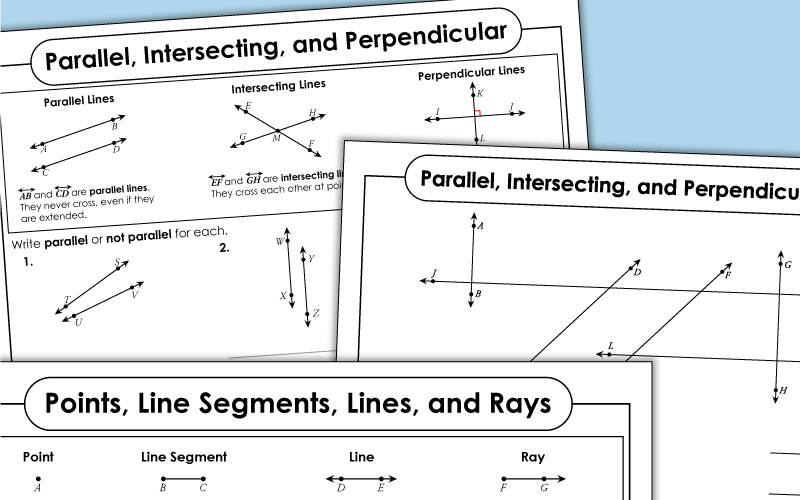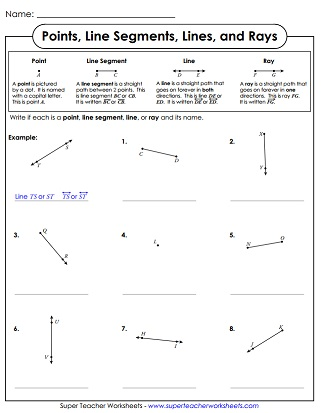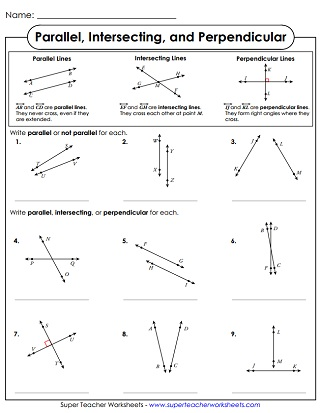# Line Segments

These worksheets help students learn about lines, line segments, rays, parallel lines, intersecting lines, and perpendicular lines.Identify and label the points, lines, line segments, and rays pictured.
Using a small street map, students must determine if each pair of streets is parallel, intersecting, or perpendicular.
Tell whether each pair of lines is parallel, intersecting, or perpendicular.
Find parallel, intersecting and perpendicular lines in the illustration.
Match the words with the definitions: line, line segment, ray, parallel lines, perpendicular lines, intersecting lines
Fill-in-the-blank questions and multiple choice questions about points, lines, rays, parallel, and perpendicular lines.
Answer the questions about line segments, rays, parallel lines, and perpendicular lines.
For this activity, students must choose the correct definition for the words line, line segment, ray, point, parallel, intersecting, and perpendicular. They also draw each item.
Plotting Points

Worksheets for teaching students to plot ordered pairs on coordinate grids

Angle Worksheets

Worksheets with angles and triangles

Math Worksheets

Full math worksheet index

## Sample Worksheet ImagesMy Account
Site Information